### Home > AC > Chapter 7 > Lesson 7.1.5 > Problem7-48

7-48.
1. Consider this system of equations: Homework Help ✎

y = 2x − 8

y =1. Use your knowledge of y = mx + b to graph the lines without tables.

2. Use the graph to find the point of intersection.

3. Confirm this point of intersection by solving the system algebraically.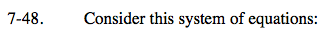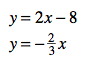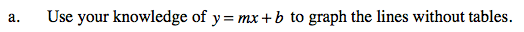Remember:
b = the y-intercept
m = the slope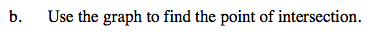The point of intersection is (3, −2). Where is this point on the graph?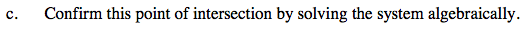Use the Equal Values Method.